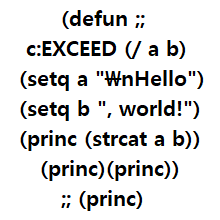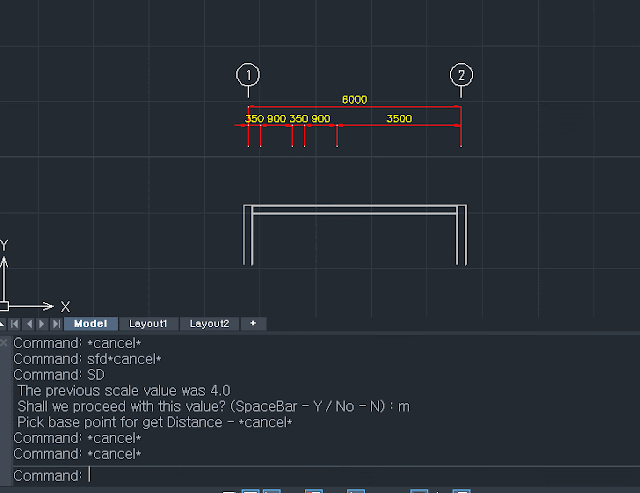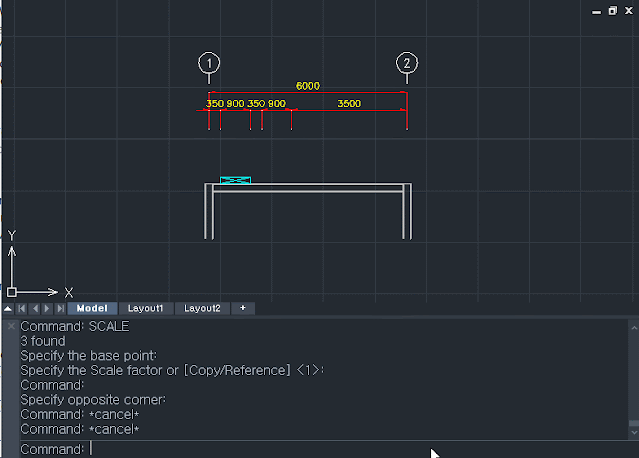# Simple Scaled Drawing## Recommended PostsPosted (edited)

```; Scaled Drawing - 2022.05.09 exceed
; SD - Scaled Distance
; SL - Scaled Line
; SP - Scaled LWpolyline
; SR - Scaled Rectangle
; SM - Scaled Move
; SCOPY - Scaled Copy
; SCIRCLE - Scaled Circle
; SWD - Scale With Dimension

(defun c:SL ( / *error* rescaleyn pt1 pt2 ang dist )
(setvar 'cmdecho 0)
(LM:startundo (LM:acdoc))
;error control
(defun *error* ( msg )
(LM:endundo (LM:acdoc))
(if (not (wcmatch (strcase msg t) "*break,*cancel*,*exit*"))
(princ (strcat "\n Error: " msg))
)
(setvar 'cmdecho 1)
(princ)
)

(setq scpt1 (getpoint "\n Pick 1st point for Scale - "))
(princ scpt1)
(setq scpt2 (getpoint scpt1 "\n Pick 2nd point for Scale - "))
(princ scpt2)
(setq xdist (- (car scpt2) (car scpt1)))
(princ "\n distnace - X : ")
(princ xdist)
(princ ", Y : ")
(princ ydist)
(setq scaleanswer (getreal "\n How long is this Length Actually? "))
(setq scdist (distance scpt1 scpt2))
slscale
)

(if (or (= slscale nil) (= slscale 0) (= slscale ""))
(progn
(setq slscale (ex:getscale))
);end of progn
(progn
(princ "\n The previous scale value was ")
(princ slscale)
(setq rescaleyn (getstring " Shall we proceed with this value? (SpaceBar - Y / No - N) : "))
(if (= (strcase rescaleyn) "N")
(progn
(setq slscale (ex:getscale))
);end of progn
);end of if
);end of progn
);end of if

(setq pt1 (getpoint "\n Pick base point for Line - "))
(princ pt1)
(princ "\n Scale Value : ")
(princ slscale)
(while (setq pt2 (getpoint pt1 "\n Pick next point for Line - "))
(princ pt2)
(setq ang (angle pt1 pt2))
(setq dist (* (distance pt1 pt2) slscale))
(setq pt2 (polar pt1 ang dist))
(entmake (list (cons 0 "LINE") (cons 10 pt1) (cons 11 pt2)))
(setq pt1 pt2)
);end of while

(LM:endundo (LM:acdoc))
(setvar 'cmdecho 1)
(princ)
);end of defun

(defun c:SM ( / *error* rescaleyn pt1 pt2 ang dist mss )
(setvar 'cmdecho 0)
(LM:startundo (LM:acdoc))
;error control
(defun *error* ( msg )
(LM:endundo (LM:acdoc))
(if (not (wcmatch (strcase msg t) "*break,*cancel*,*exit*"))
(princ (strcat "\n Error: " msg))
)
(setvar 'cmdecho 1)
(princ)
)

(setq scpt1 (getpoint "\n Pick 1st point for Scale - "))
(princ scpt1)
(setq scpt2 (getpoint scpt1 "\n Pick 2nd point for Scale - "))
(princ scpt2)
(setq xdist (- (car scpt2) (car scpt1)))
(princ "\n distnace - X : ")
(princ xdist)
(princ ", Y : ")
(princ ydist)
(setq scaleanswer (getreal "\n How long is this Length Actually? "))
(setq scdist (distance scpt1 scpt2))
slscale
)

(if (or (= slscale nil) (= slscale 0) (= slscale ""))
(progn
(setq slscale (ex:getscale))
);end of progn
(progn
(princ "\n The previous scale value was ")
(princ slscale)
(setq rescaleyn (getstring " Shall we proceed with this value? (SpaceBar - Y / No - N) : "))
(if (= (strcase rescaleyn) "N")
(progn
(setq slscale (ex:getscale))
);end of progn
);end of if
);end of progn
);end of if

(princ "\n Select Objects to move - ")
(setq mss (ssget ":L"))

(setq pt1 (getpoint "\n Pick base point for move - "))
(princ pt1)
(princ "\n Scale Value : ")
(princ slscale)
(setq pt2 (getpoint pt1 "\n Pick next point for move - "))
(princ pt2)
(setq ang (angle pt1 pt2))
(setq dist (* (distance pt1 pt2) slscale))
(setq pt2 (polar pt1 ang dist))
(command "_.MOVE" mss "" pt1 pt2)
(LM:endundo (LM:acdoc))
(setvar 'cmdecho 1)
(princ)
);end of defun

(defun c:SCOPY ( / *error* rescaleyn pt1 pt2 ang dist mss )
(setvar 'cmdecho 0)
(LM:startundo (LM:acdoc))
;error control
(defun *error* ( msg )
(LM:endundo (LM:acdoc))
(if (not (wcmatch (strcase msg t) "*break,*cancel*,*exit*"))
(princ (strcat "\n Error: " msg))
)
(setvar 'cmdecho 1)
(princ)
)

(setq scpt1 (getpoint "\n Pick 1st point for Scale - "))
(princ scpt1)
(setq scpt2 (getpoint scpt1 "\n Pick 2nd point for Scale - "))
(princ scpt2)
(setq xdist (- (car scpt2) (car scpt1)))
(princ "\n distnace - X : ")
(princ xdist)
(princ ", Y : ")
(princ ydist)
(setq scaleanswer (getreal "\n How long is this Length Actually? "))
(setq scdist (distance scpt1 scpt2))
slscale
)

(if (or (= slscale nil) (= slscale 0) (= slscale ""))
(progn
(setq slscale (ex:getscale))
);end of progn
(progn
(princ "\n The previous scale value was ")
(princ slscale)
(setq rescaleyn (getstring " Shall we proceed with this value? (SpaceBar - Y / No - N) : "))
(if (= (strcase rescaleyn) "N")
(progn
(setq slscale (ex:getscale))
);end of progn
);end of if
);end of progn
);end of if

(princ "\n Select Objects to move - ")
(setq mss (ssget ":L"))

(setq pt1 (getpoint "\n Pick base point for move - "))
(princ pt1)
(princ "\n Scale Value : ")
(princ slscale)
(setq pt2 (getpoint pt1 "\n Pick next point for move - "))
(princ pt2)
(setq ang (angle pt1 pt2))
(setq dist (* (distance pt1 pt2) slscale))
(setq pt2 (polar pt1 ang dist))
(command "_.COPY" mss "" pt1 pt2)
(LM:endundo (LM:acdoc))
(setvar 'cmdecho 1)
(princ)
);end of defun

(defun c:SP ( / *error* rescaleyn pt1 pt2 ang dist )
(setvar 'cmdecho 0)
;error control
(defun *error* ( msg )
(LM:endundo (LM:acdoc))
(if (not (wcmatch (strcase msg t) "*break,*cancel*,*exit*"))
(princ (strcat "\n Error: " msg))
)
(setvar 'cmdecho 1)
(princ)
)

(setq scpt1 (getpoint "\n Pick 1st point for Scale - "))
(princ scpt1)
(setq scpt2 (getpoint scpt1 "\n Pick 2nd point for Scale - "))
(princ scpt2)
(setq xdist (- (car scpt2) (car scpt1)))
(princ "\n distnace - X : ")
(princ xdist)
(princ ", Y : ")
(princ ydist)
(setq scaleanswer (getreal "\n How long is this actually? "))
(setq scdist (distance scpt1 scpt2))
slscale
)

(if (or (= slscale nil) (= slscale 0) (= slscale ""))
(progn
(setq slscale (ex:getscale))
);end of progn
(progn
(princ "\n The previous scale value was ")
(princ slscale)
(setq rescaleyn (getstring " Shall we proceed with this value? (SpaceBar - Y / No - N) : "))
(if (= (strcase rescaleyn) "N")
(progn
(setq slscale (ex:getscale))
);end of progn
);end of if
);end of progn
);end of if
(setq pt1 (getpoint "\n Pick base point for PolyLine - "))
(princ pt1)
(princ "\n Scale Value : ")
(princ slscale)
(while (setq pt2 (getpoint pt1 "\n Pick next point for PolyLine - "))
(princ pt2)
(setq ang (angle pt1 pt2))
(setq dist (* (distance pt1 pt2) slscale))
(setq pt2 (polar pt1 ang dist))
(entmake (list (cons 0 "LWPOLYLINE") (cons 100 "AcDbEntity") (cons 67 0) (cons 100 "AcDbPolyline") (cons 90 2) (cons 70 0) (cons 43 0) (cons 38 0) (cons 39 0) (cons 10 (list (car pt1) (cadr pt1))) (cons 40 0) (cons 41 0) (cons 42 0) (cons 91 0) (cons 10 (list (car pt2) (cadr pt2))) (cons 40 0) (cons 41 0) (cons 42 0) (cons 91 0)))
(if (> (sslength ss) 1)
(progn
(command "pedit" "multiple" ss "" "j" 0 nil)
)
)
;(setq ent1 ent2)
(setq pt1 pt2)
);end of while

(LM:endundo (LM:acdoc))
(setvar 'cmdecho 1)
(princ)
);end of defun

(defun c:SCIRCLE ( / *error* rescaleyn pt1 pt2 ang dist )
(setvar 'cmdecho 0)
(LM:startundo (LM:acdoc))
;error control
(defun *error* ( msg )
(LM:endundo (LM:acdoc))
(if (not (wcmatch (strcase msg t) "*break,*cancel*,*exit*"))
(princ (strcat "\n Error: " msg))
)
(setvar 'cmdecho 1)
(princ)
)

(setq scpt1 (getpoint "\n Pick 1st point for Scale - "))
(princ scpt1)
(setq scpt2 (getpoint scpt1 "\n Pick 2nd point for Scale - "))
(princ scpt2)
(setq xdist (- (car scpt2) (car scpt1)))
(princ "\n distnace - X : ")
(princ xdist)
(princ ", Y : ")
(princ ydist)
(setq scaleanswer (getreal "\n How long is this Length Actually? "))
(setq scdist (distance scpt1 scpt2))
slscale
)

(if (or (= slscale nil) (= slscale 0) (= slscale ""))
(progn
(setq slscale (ex:getscale))
);end of progn
(progn
(princ "\n The previous scale value was ")
(princ slscale)
(setq rescaleyn (getstring " Shall we proceed with this value? (SpaceBar - Y / No - N) : "))
(if (= (strcase rescaleyn) "N")
(progn
(setq slscale (ex:getscale))
);end of progn
);end of if
);end of progn
);end of if

(while (setq pt1 (getpoint "\n Pick center point for Circle - "))
(princ pt1)
(princ "\n Scale Value : ")
(princ slscale)
(setq pt2 (getpoint pt1 "\n Pick next point for Line - "))
(princ pt2)
(setq ang (angle pt1 pt2))
(setq dist (* (distance pt1 pt2) slscale))
(setq pt2 (polar pt1 ang dist))
(entmake (list (cons 0 "CIRCLE") (cons 10 pt1) (cons 40 (/ dist 2))))
);end of while

(LM:endundo (LM:acdoc))
(setvar 'cmdecho 1)
(princ)
);end of defun

(defun c:SR ( / *error* rescaleyn pt1 pt2 ang dist width height )
(setvar 'cmdecho 0)
(LM:startundo (LM:acdoc))
;error control
(defun *error* ( msg )
(LM:endundo (LM:acdoc))
(if (not (wcmatch (strcase msg t) "*break,*cancel*,*exit*"))
(princ (strcat "\n Error: " msg))
)
(setvar 'cmdecho 1)
(princ)
)

(setq scpt1 (getpoint "\n Pick 1st point for Scale - "))
(princ scpt1)
(setq scpt2 (getpoint scpt1 "\n Pick 2nd point for Scale - "))
(princ scpt2)
(setq xdist (- (car scpt2) (car scpt1)))
(princ "\n distnace - X : ")
(princ xdist)
(princ ", Y : ")
(princ ydist)
(setq scaleanswer (getreal "\n How long is this Length Actually? "))
(setq scdist (distance scpt1 scpt2))
slscale
)

(if (or (= slscale nil) (= slscale 0) (= slscale ""))
(progn
(setq slscale (ex:getscale))
);end of progn
(progn
(princ "\n The previous scale value was ")
(princ slscale)
(setq rescaleyn (getstring " Shall we proceed with this value? (SpaceBar - Y / No - N) : "))
(if (= (strcase rescaleyn) "N")
(progn
(setq slscale (ex:getscale))
);end of progn
);end of if
);end of progn
);end of if

(while (setq pt1 (getpoint "\n Pick base point for Rectangle (left lower point) - "))
(princ pt1)
(princ "\n Scale Value : ")
(princ slscale)
;(setq pt2 (getpoint pt1 "\n Pick next point for Rectangle - "))
;(princ pt2)
(setq width (getreal "\n Input Width : "))
(setq height (getreal "\n Input Height "))
(setq pt2 (list (+ (car pt1) width) (+ (cadr pt1) height) (caddr pt1)))
(setq ang (angle pt1 pt2))
(setq dist (* (distance pt1 pt2) slscale))
(setq pt2 (polar pt1 ang dist))
(entmake (list (cons 0 "LWPOLYLINE") (cons 100 "AcDbEntity") (cons 67 0) (cons 100 "AcDbPolyline") (cons 90 2) (cons 70 1) (cons 43 0) (cons 38 0) (cons 39 0) (cons 10 (list (car pt1) (cadr pt1))) (cons 40 0) (cons 41 0) (cons 42 0) (cons 91 0) (cons 10 (list (car pt1) (cadr pt2))) (cons 40 0) (cons 41 0) (cons 42 0) (cons 91 0) (cons 10 (list (car pt2) (cadr pt2))) (cons 40 0) (cons 41 0) (cons 42 0) (cons 91 0) (cons 10 (list (car pt2) (cadr pt1))) (cons 40 0) (cons 41 0) (cons 42 0) (cons 91 0)))
);end of while

(LM:endundo (LM:acdoc))
(setvar 'cmdecho 1)
(princ)
);end of defun

(defun c:SD ( / *error* rescaleyn pt1 pt2 ang dist )
(setvar 'cmdecho 0)
(LM:startundo (LM:acdoc))
;error control
(defun *error* ( msg )
(LM:endundo (LM:acdoc))
(if (not (wcmatch (strcase msg t) "*break,*cancel*,*exit*"))
(princ (strcat "\n Error: " msg))
)
(setvar 'cmdecho 1)
(princ)
)

(setq scpt1 (getpoint "\n Pick 1st point for Scale - "))
(princ scpt1)
(setq scpt2 (getpoint scpt1 "\n Pick 2nd point for Scale - "))
(princ scpt2)
(setq xdist (- (car scpt2) (car scpt1)))
(princ "\n distnace - X : ")
(princ xdist)
(princ ", Y : ")
(princ ydist)
(setq scaleanswer (getreal "\n How long is this Length Actually? "))
(setq scdist (distance scpt1 scpt2))
slscale
)

(if (or (= slscale nil) (= slscale 0) (= slscale ""))
(progn
(setq slscale (ex:getscale))
);end of progn
(progn
(princ "\n The previous scale value was ")
(princ slscale)
(setq rescaleyn (getstring " Shall we proceed with this value? (SpaceBar - Y / No - N) : "))
(if (= (strcase rescaleyn) "N")
(progn
(setq slscale (ex:getscale))
);end of progn
);end of if
);end of progn
);end of if

(while (setq pt1 (getpoint "\n Pick base point for get Distance - "))
(princ pt1)
(princ "\n Scale Value : ")
(princ slscale)
(setq pt2 (getpoint pt1 "\n Pick next point for get Distance - "))
(princ pt2)
(setq ang (angle pt1 pt2))
(setq sxdist (/ (- (car pt2) (car pt1)) slscale))
(princ "\n distance - X : ")
(princ sxdist)
(princ ", Y : ")
(princ sydist)
(setq dist (/ (distance pt1 pt2) slscale))
(princ "\n Scaled Distance : ")
(princ dist)

);end of while

(LM:endundo (LM:acdoc))
(setvar 'cmdecho 1)
(princ)
);end of defun

(defun c:SWD ( / *error* slscale rescaleyn ss index ename obj type scfactor lnscfactor bp )
(setvar 'cmdecho 0)
(LM:startundo (LM:acdoc))
;error control
(defun *error* ( msg )
(LM:endundo (LM:acdoc))
(if (not (wcmatch (strcase msg t) "*break,*cancel*,*exit*"))
(princ (strcat "\n Error: " msg))
)
(setvar 'cmdecho 1)
(princ)
)

(setq scpt1 (getpoint "\n Pick 1st point for Scale - "))
(princ scpt1)
(setq scpt2 (getpoint scpt1 "\n Pick 2nd point for Scale - "))
(princ scpt2)
(setq xdist (- (car scpt2) (car scpt1)))
(princ "\n distnace - X : ")
(princ xdist)
(princ ", Y : ")
(princ ydist)
(setq scaleanswer (getreal "\n How long is this Length You Want? "))
(setq scdist (distance scpt1 scpt2))
slscale
)

(setq ss (ssget ":L"))

(if (or (= slscale nil) (= slscale 0) (= slscale ""))
(progn
(setq slscale (ex:getreversescale))
);end of progn
(progn
(princ "\n The previous scale value was ")
(princ slscale)
(setq rescaleyn (getstring " Shall we proceed with this value? (SpaceBar - Y / No - N) : "))
(if (= (strcase rescaleyn) "N")
(progn
(setq slscale (ex:getreversescale))
);end of progn
);end of if
);end of progn
);end of if

(setq bp (getpoint "\n Pick base point for Scale - "))
(command "_.SCALE" ss "" bp slscale)

(setq ssl (sslength ss))
(setq index 0)

(repeat ssl
(setq ename (ssname ss index))
(setq obj (vlax-ename->vla-object ename))
(setq type (vlax-get-property obj 'EntityName))
(cond
((or (= type "AcDbRotatedDimension") (= type "AcDbAlignedDimension"))
;(princ "\n it's dimension")
(setq scfactor (atof (vl-princ-to-string (vlax-get-property obj 'ScaleFactor))))
(setq lnscfactor (atof (vl-princ-to-string (vlax-get-property obj 'LinearScaleFactor))))
(princ "\n scfactor - ")
(princ scfactor)
(princ " lnscfactor - ")
(princ lnscfactor)
(vla-put-scalefactor obj (* scfactor slscale))
(vla-put-linearscalefactor obj (/ lnscfactor slscale))
)

);end of cond

(setq index (+ index 1))
);end of repeat

(LM:endundo (LM:acdoc))
(setvar 'cmdecho 1)
(princ)
);defun

;; Active Document  -  Lee Mac
;; Returns the VLA Active Document Object

(defun LM:acdoc nil
(eval (list 'defun 'LM:acdoc 'nil (vla-get-activedocument (vlax-get-acad-object))))
(LM:acdoc)
)

;; Start Undo  -  Lee Mac
;; Opens an Undo Group.

(defun LM:startundo ( doc )
(LM:endundo doc)
(vla-startundomark doc)
)

;; End Undo  -  Lee Mac
;; Closes an Undo Group.

(defun LM:endundo ( doc )
(while (= 8 (logand 8 (getvar 'undoctl)))
(vla-endundomark doc)
)
)```

Command List

SD - Scaled Distance
SL - Scaled Line
SP - Scaled LWpolyline
SR - Scaled Rectangle
SM - Scaled Move
SCOPY - Scaled Copy
SCIRCLE - Scaled Circle

SWD - Scale with DimensionSD, SL, SRSM, SCOPY

- how to use

First, specify two points in the view, and enter how long the distance is in the view.

Then draw line, polyline, rectangle, circle or move, copy, measure distance.

After specifying the base point, get the angle from the next getdist state

and get the distance as user keyboard input.

If you enter a scale that is different from the actual scale once, the drawing is made with that scale.

If 4 is recognized as 2, If you draw 2, you get 4, If you draw 4, you get 8.

this lisp used when there are 2 or more scales in one model space.

ex) main plan view 1:1500, section view 1:100, enlarged view 1:200, detached view 1:50.

(I know it's smarter to use paper space)

+

Scale the already properly created section drawings and dimensions together. (The scale factor and linear scale factor are adjusted.)

Edited by exceed
##### Share on other sitesI am not sure what it is your trying to do the one rule nearly everybody pushes is draw real size not scaled. That is why layouts have MVIEW.

•1
##### Share on other sitesthere is wrong industries in world. unfortunately.

in my work also use mview or xclips but in very small project only. like 1 or 2 buildings.

##### Share on other sites## Join the conversation

You can post now and register later. If you have an account, sign in now to post with your account.
Note: Your post will require moderator approval before it will be visible.×   Pasted as rich text.   Restore formatting

Only 75 emoji are allowed.